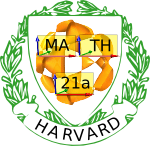Fall 2006

# Mathematics Math21a Fall 2006

## Multivariable Calculus

Office: SciCtr 434
Email: knill@math.harvard.edu
Harvard Mathematics

 Weekly checklists: "Somewhere, over the rainbow ..."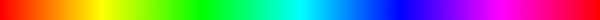Week1 Week2 Week3 Week4 Week5 Week6 Week7 Week8 Week9 Week10 Week11 Week12

 Send questions of potential general interest to math21a@fas.harvard.edu.
 Question: When do we write int F(r(u,v)) . ru x rv du dv and when do wr write int F(r(u,v)) . n |ru x rv du dv for the flux integral? Answer: The two expressions are the same. Our book uses the second notation with the unit normal vector n. There are several reasons, why it is better to avoid it: it is a more complicated notation it seduces you to compute the unit vector n which is not necessary. It does not make sense if the surface has places, where the vector ru x rv is zero. The first notation still works.
 Question: How do you define parallel vectors? Do they have to run in the same direction? or can they run in opposite directions and still be parallel? Answer: Parallel vectors can also point in opposite directions. The vector (2,3,4) is parallel to (-8,-12,-16). The zero vector is parallel to any vector. Two vectors are parallel if and only if their cross product vanishes.
Question: I am trying to do Episode 4 practice exam, and I'm confused about problem 5: how do you get f(x,y)=x^4+10x^2+25+y^4-2y^2+1? I can see that F(x,y)= is a gradient field, but I don't get where the f(x,y) comes from. Answer: you have to extremize the length of the vector field, not the potential To extremize the length, you can also extremize the square of the length. While you are right that the vector field is a gradient field, this is irrelevant in this question. There are different ways how you can derive a scalar field from a vector field:

 F -> f if F = grad(f) F -> curl(F) in two dimensions F -> div(F) in any dimensions F -> |F| in any dimensions

In all these cases, the middle entry is a scalar field. In the problem of your question, we look at the length of the vector field.
 Question: In the Fridays review, in the flowchart shown, one of the boxes said to check to see if a given vector field is the curl of something else. Is there any quick method to checking that? Answer: yes, there is. If the divergence is zero everywhere in space then there the vector field is the curl of something else. There are some cool integral formulas which give you G such that F=curl(G) but they were not covered in our course. We do not assume that you know this and we would give you the vector field G. There are cases, where one can guess it like F = (0,0,2), where one could take G = (-y,x,0).
 Question: Could you clarify for what parts of the homework Mathematica usage is allowed (e.g. when should we use Mathematica or not)? Answer:You can use Mathematica for all your homework. But you have to include all steps of your computations. For example, if you use Mathematica for checking the result of an integral, you still have to include all integration steps. For example, if you compute a triple integral, we need to see how you compute the most inner integral, then the middle integral and finally the outer integral. If you draw something with Mathematica, we need to see your code. Just printing out the notebook you created is ok. It is often much easier to plot it by hand. In general, you have to acknowledge all use of Mathematica and document, what you do.
 Question: Why do you test TF questions in exams. They are often hard. Yes, we know. They are tricky but they are also fun. We want you to learn the material as efficiently as possible. There is a definition aspect (know the terms) an algorithmic aspect (compute stuff) and a conceptional aspect (understanding things) in learning. Experience shows that asking too many conceptional problems as major problems in exams can be frustrating, both for students as well as the graders. Multiple choice questions are an elegant way to include this level nevertheless. TF questions push the boundary of your knowledge, they can allow you to detect and fix misconceptions, which you would not see when practicing algorithmic tasks only. We always advise not to spend too much time with the TF problems during the exam, but to think about them well when doing the practice exams.
Question: I have trouble understanding section 13.3. Don't worry, you can skip this. Epsilon delta definitions of continuity are not very intuitive. It usually is hammered into the brains by intimidation and repetition. The mathematician Edward Nelson gave once the following example: compare the usual definition of continuity: a function f is continuous at x0 if
```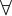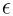> 0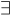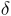> 0, so that if |x-x0|<then  |f(x)-f(x0)| <```
with the following statement:
```> 0> 0, so that if |x-x0|<then  |f(x)-f(x0)| <```Euler What does the later statement mean? --- You probably had to think hard for a while to get it. Everybody has! Try it out with your friends taking math 23a. This proves that the Weierstrass notion of continuity is unintuitive. And as usual with didactics, only experiment with real people reveals this. An epsilon-delta definition is a good working definition, if you do formal proofs, if you are a mathematician. Euler has a much better definition: f is continuous at x0, if for all x close to x0 the value f(x) is close to f(x0). It is as precise as the Weierstrass definition, if one defines "close" appropriatly. This is done in nonstandard analysis, which clears up the most dreadful parts of calculus. It never made it to the calculus curricula because it would make half of the calculus teachers oblivious ...Weierstrass
By the way, do you know the shortest joke mathematicians tell each other? Here it is:
```= 0
```
Don't think, it is funny? Well, it only works on mathematicians.
 Question: Can you explain the notations exp and log? Answer: The function exp is the exponential function. It means exp(t) = et. The function log is the logarithm function. Unlike in high schools, where one often uses ln(x) for the natural logarithm, the logarithm is so important that it is called log in most of the mathematical and scientific literature. The logarithm to the base 10 is not natural. It happend just by accident that humans have 10 fingers. Also Mathematica uses Log for the natural logaritm. The function log is the inverse of exp: one has ``` exp(log(x)) = x log(exp(x)) = x ``` Note that d/dx log(x) = 1/x for positive x and d/dx exp(x) = exp(x).
 Question: I don't understand what the ways of converting back and forth from parametric and implicit are. "Read off the radius" or "know it" make no sense to me. Also, maybe you could conceptually explain the ways that parametric and implicit are converted back and forth for the other surfaces as well. Answer: There are not many surfaces, where you can switch forth and back from implicit to parametric. In general, it is not possible. These are two different worlds and going from one to the other is not easy. There are no general tools to do that. What we can do are 1) Spheres and general quadrics 2) surfaces of revolution 3) planes, 4) graphs. I don't know for example, how to parametrize general Kummer surface. One could solve for one of the variables but then one would only parametrize part of the surface which are graphs. So, in general we are completely helpless in finding parametrizations of implicit surfaces and finding an implicit description of parametric surfaces.
Question: Do we need a graphing calculator for the first HW problem in the 4th week? Answer: Absolutely not. This was just mentioned if you want to have som fun with a toy. You have just to write down an equation of a surface in cylindrical coordinates and an equation of a surface in spherical coordinates. No graphing calculator is needed for that. Now, you have to be able to draw this surface. For example, your homework solution to the two problems could look as follows, if it were not too simple.

 PROBLEM A. My equation is r = 1. This is a cylinder. Here is a picture. PROBLEM B. My equation is rho =1. This is a sphere. Here is a picture.
Start with simple things like r = z (a cone) or rho = 1/cos(phi) which is the plane z = 1. If you use too complicated formulas, you will no more be able to analyze or draw the surfaces.
 Question: Can the radius r in polar coordinates become negative? Answer: many calculus books including our book allow that when drawing polar curves like r = sin(theta). In general, it is better to avoid negative radii. The radius is the distance to the origin. If one allows negative r, then one adds more ambiguity into the point (-1,0) could be written as (r,theta) = (1,Pi) or (-1,0).
 Question: I have some problems with problem 32 for Monday October 16. Answer: It is best to look at the normal vector n = (-2t,1) to the curve and not the tangent vector (as the hint says). The reflected vector of the vector v = (0,-1) is with the projection vector Pn(v) given by ```w = v-2 Pn(v) ``` Now the computation is easier: ```r(t) = ( t , t^2 ) point on parabola r'(t) = ( 1 , 2t ) tangent vector n(t) = ( -2t, 1 ) normal vector v = ( 0, -1 ) incoming vector w = v-2 (v. n ) n/(1+4t2) = ( 0, -1) +2 ( -2t/(1+4t2),1/(1+4t2) ) = (-4t/(1+4t2),-1+2/(1+4t2)) reflected vector ``` reflected ray parametrization ```(t,t2) + s ( -4t/(1+4t2),-1+2/(1+4t2) ) = (t - 4 t s/(1+4t2), t2-s+2s/(1+4t2) ) ``` Look for the s for which the first coordinate is zero and show that then the y coordinate is constant independent of t.
 Question: Do we have to know section 12.5 in the book? No.
 Question: We have covered curvature only for a very short time in class. What do we have to know about curvature in the exam? While the topic of curvature is exciting, we only scratched the surface. You do not have to know curvature formulas nor know about osculating circles. We recommend however that you read about it. It is a nice topic. By the way, in order to compute curvature like in the homework problem, it can pay off to plug in numbers early. If you want to compute the curvature at a specific point, it is enough to compute the velocity vector r' and acceleration vector r'' at the point and then compute the curvature with |r' x r''|/ |r'|3 after you have plugged in the time t. If you carry along the full computation with the variable t until the end, you might get into a terrible mess.
 Question: Q; On problem 42 in section 12.3 of the Math 21a homework, the the arc length function is far too complex. Mathematica gives a nasty solution. Humans are still better than machines! The core problem is that Mathematica does not simplify Sqrt[x^2] to x. Mathematica produces from ```r[t_]:= { Cos[t]^3, Sin[t]^3 }; Integrate[Sqrt[r'[s].r'[s]],{s,0,t}] ``` the output ```(3*(Sqrt[t^2] - t*Cot[2*t]*Sqrt[Sin[2*t]^2]))/(4*t) ``` which is of course equal to (3/4) cos(2t) but Mathematica does not simplify (because taking square roots is a source of errors). A human can simplify because sigma(t) is nonnegative ``` r'(t) = (- 3 cos^2(t) sin(t), 3 sin^2(t) cos(t) ) |r'(t)| = 3 sin(t) cos(t) = (3/2) sin(2t) sigma(t) = (3/4) cos(2t) ```
 Question: Q; I am looking at section 12.3 and am confused about some symbols the book is using. On page 80, under the definition, it says u |--> r(t0) + ur'(t0). What does the arrow |--> mean? I've never seen that notation, and it comes up in #50 of our problem set. Is it just another way of defining a curve parameterized by a vector? If so, would that mean that in #50, it's just talking about two curves C1(t)= and C2(t)=<3t+1, 2t, t^2+t-1>? Instead of f(x) = sin(x), one also uses the notation x |--> sin(x) This allows the autors of the book to avoid introducing a new function on page 80. The tangent line in this case is again a parametrized curve, one can write it for example as ``` R(u) = r(t0) + u r'(t0) ``` For the tangent curve, one has taken a new parameter u. It is an other parameter than t.
 Question: Q; There are some problems in the book which are labeled "Computer exercices" We rarely assign those, but if you you have one, why not get started already with Mathematica and do it with Mathematica. You should also be able to do it with a calculator, but we strongly suggest to get away from calculators and use a more powerful computer algebra system.
 Question: Q; The coop is out of text books. Sorry about the invonvenience. We might post the problems to first week online and investigate why there are not enough copies. It is sold on online shops like Amazon but we don't know how long it takes to ship at the moment.
 Question: Q: Why do we use an other book this semester? Answer: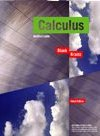There are several reasons to try an alternative to Stewart: Solutions for Stewarts book are now widely available online. This happend especially after CDs of the solutions were available and many students had access to solution books. The prize of Stewart is unreasonable. The new book of Blanck and Krantz contains all we need and is half the prize.